# MonoGame: Rectangles and Collision

By Stephen Armstrong // January 7th, 2020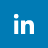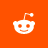## Learn how to create, move, resize, and detect collision on Rectangles.

The following C# code will tell you how to use Rectangles for layout and collision detection in a MonoGame project.

Rectangles are basic shapes that provide core functionality in your game.

They are mainly used for positioning and collision detection.

## Creating a Rectangle

The easiest way to create a Rectangle is to set its position and size in the constructor:

• // Create a rectangle.
• int x = 10;
• int y = 20;
• int width = 30;
• int height = 40;
• Rectangle rectangle = new Rectangle(x, y, width, height);
• System.Console.WriteLine("New Rectangle: {0}", rectangle);

This creates a Rectangle that’s 30 pixels wide, 40 pixels tall. The Rectangle is positioned at 10 x 20.

## Getting Rectangle Boundaries and Center

The following Properties can be used to get their respective values.

Name Type Description
.Left int The Rectangle's left position (X)
.Right int The Rectangle's right position (X + Width)
.Top int The Rectangle's top position (Y)
.Bottom int The Rectangle's bottom position (Y + Height)
.Center Point The middle of the Rectangle

You can test these Properties by using the following code:

• // Display the boundaries of the Rectangle.
• System.Console.WriteLine("Rectangle left: {0}", rectangle.Left);
• System.Console.WriteLine("Rectangle right: {0}", rectangle.Right);
• System.Console.WriteLine("Rectangle top: {0}", rectangle.Top);
• System.Console.WriteLine("Rectangle bottom: {0}", rectangle.Bottom);
• // Display the center point of the Rectangle.
• System.Console.WriteLine("Rectangle center: {0}", rectangle.Center);

When you run this code you will see the following in the Output: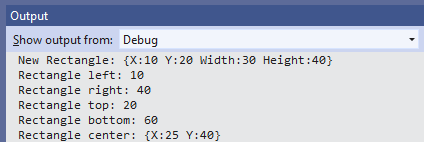## Moving a Rectangle

The simplest way to move a rectangle is to increase/decrease its X and/or Y values.

• // Move the Rectangle one pixel to the right.
• rectangle.X++;
• // Move the Rectangle ten pixels up.
• rectangle.Y -= 10;

Another way is to use .Offset():

• // Move the Rectangle 50 pixels left and 100 pixels down.
• rectangle.Offset(-50, 100);

## Resizing a Rectangle

A Rectangle can be resized by changing its Width and/or Height values.

• // Increase the width of the Rectangle by 20 pixels.
• rectangle.Width += 20;
• // Decrease the height of the Rectangle by 5 pixels.
• rectangle.Height -= 5;

Please keep in mind that the X and Y values of the Rectangle will remain the same if you modify the Width and Height.

To maintain the same center point of the Rectangle while resizing, use .Inflate()

• // Increase the Rectangle's Width by 50 pixels, and the Height by 200 pixels.
• // This will move the Rectangle 25 pixels to the left and 100 pixels up.
• rectangle.Inflate(25, 100);

Please note when resizing Rectangles that they support negative values. Do not let the width and height of Rectangles become negative values, otherwise it will break functionality.

## Intersect() and Contains()

These two Methods are extremely important for your game, as they enable collision detection.

Intersect checks to see if one Rectangle comes into contact with another Rectangle.

Contains checks to see if another Rectangle, Point, or Vector2 is fully within a Rectangle.

The following example demonstrates many factors – including basic motion, collision detection, and color changing.

Go to Game1 and add the following fields:

• // A single-pixel texture
• Texture2D pixel;
• // A large rectangle
• Rectangle bigRectangle;
• // A small rectangle
• Rectangle smallRectangle;
• // The color of the small rectangle
• Color smallRectangleColor;
• // Controls the movement of the smallRectangle
• bool smallRectangleMovesLeft;

Now go to LoadContent() and set values for these fields:

• /// Create the single-pixel texture
• pixel = new Texture2D(GraphicsDevice, 1, 1);
• pixel.SetData<Color>(new Color[] { Color.White });
• /// Create the rectangles
• bigRectangle = new Rectangle(200, 100, 300, 150);
• smallRectangle = new Rectangle(600, 150, 50, 50);
• /// Set a default color for the smallRectangle
• smallRectangleColor = Color.White;

Now go to Draw(), and add the following code to draw both Rectangles. Don’t forget to Begin() and End() the spriteBatch!

• // Draw the big black rectangle
• spriteBatch.Draw(pixel, bigRectangle, Color.Black);
• // Draw the small rectangle that changes color on collision
• spriteBatch.Draw(pixel, smallRectangle, smallRectangleColor);

If you run the code now you should see a large black rectangle and a smaller white one:Now we go to Update() and add the following logic. This will make the smallRectangle bounce across the screen.

• // If smallRectangleMovesLeft is true, then smallRectangle moves to the left.
• if (smallRectangleMovesLeft)
• {
• // If the smallRectangle hits the left edge of the screen then it should change to move right.
• if (smallRectangle.Left == 0)
• {
• smallRectangleMovesLeft = false;
• }
• // Otherwise it should move two pixels to the left.
• else
• {
• smallRectangle.X -= 2;
• }
• }
• else
• {
• // If the smallRectangle hits the right edge of the screen then it should change to move left.
• if (smallRectangle.Right == graphics.PreferredBackBufferWidth)
• {
• smallRectangleMovesLeft = true;
• }
• // Otherwise it should move two pixels to the right.
• else
• {
• smallRectangle.X += 2;
• }
• }

Run the code now and you will see the following:Just beneath this code, add the following. This code is extremely basic collision detection code that will change the color of the smallRectangle to red if it is fully inside the bigRectangle, to orange if it simply intersects the bigRectangle, and to white is there is no collision.

• // If the bigRectangle contains the smallRectangle, the smallRectangle should turn red.
• if (bigRectangle.Contains(smallRectangle))
• {
• smallRectangleColor = Color.Red;
• }
• else
• {
• // If the bigRectangle does not contain the smallRectangle but intersects it, the smallRectangle should turn orange.
• if (bigRectangle.Intersects(smallRectangle))
• {
• smallRectangleColor = Color.Orange;
• }
• // If the bigRectangle neither contains or intersects the smallRectangle, the smallRectangle should turn white.
• else
• {
• smallRectangleColor = Color.White;
• }
• }

If you run the code, you will see the smallRectangle bounce left to right – and changing colors when it is contained by or intersects the bigRectangle.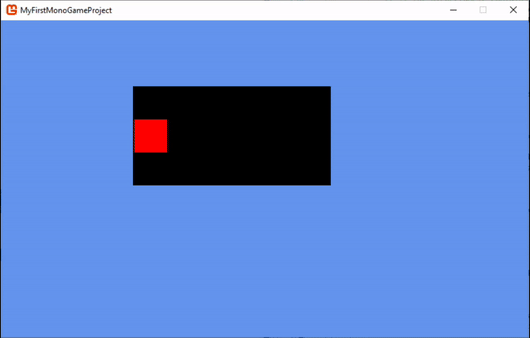In future articles I will cover collision detection in greater detail.

< Go back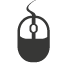Welcome to Industrian.net!

On this website you'll find more information about our games, and also some tutorials for you to start making games of your own! You can also follow us on various social platforms!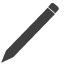Popular PostsLatest Posts